# 用Python做个海量小姐姐素描图

• 第一部分介绍两种使用python生成素描画的思路

• 第二部分介绍如何批量获取素描画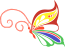1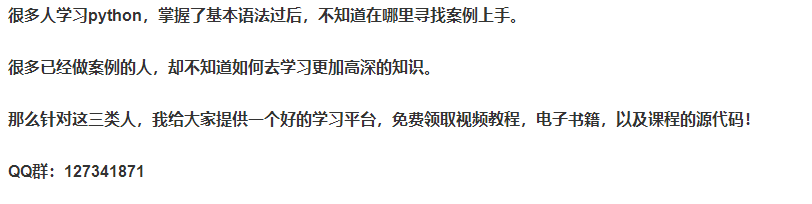### 1）漫画风格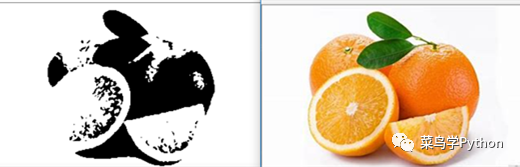``````img_rgb = cv2.imread(src_image)
img_gray = cv2.cvtColor(img_rgb, cv2.COLOR_RGB2GRAY)````````````img_edge = cv2.adaptiveThreshold(img_gray, 255,
cv2.THRESH_BINARY, blockSize=3, C=2)````````cv2.imwrite(dst_image, img_edge)````img_gray = cv2.medianBlur(img_gray, 5)``### 2）写实风格

``````img_blur = cv2.GaussianBlur(img_gray, ksize=(21, 21),
sigmaX=0, sigmaY=0)````````cv2.divide(img_gray, img_blur, scale=255)``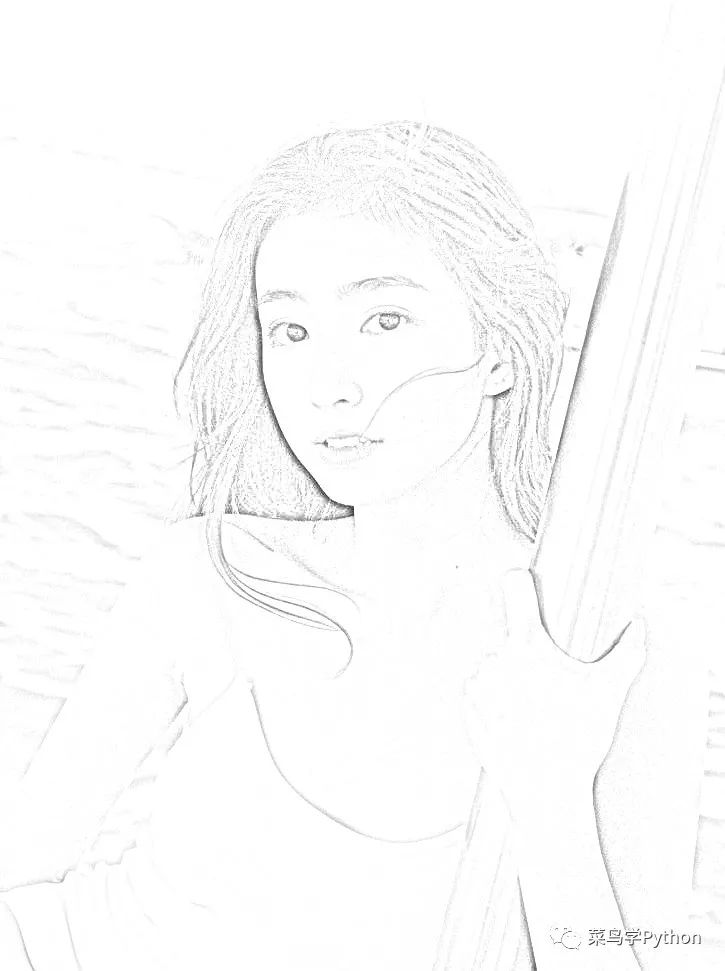2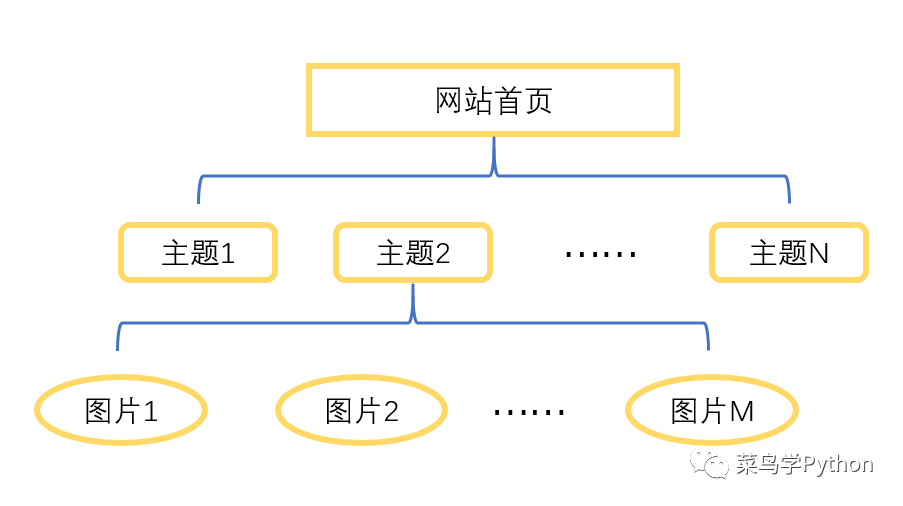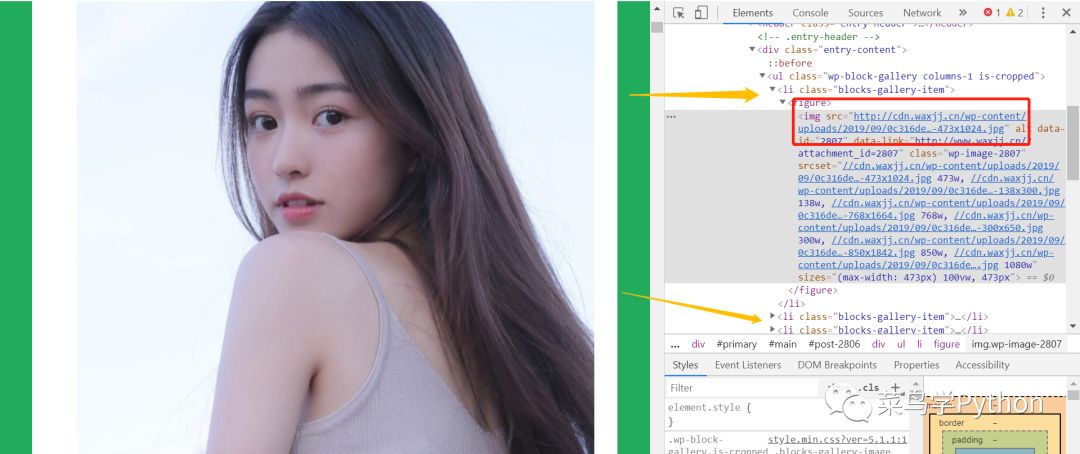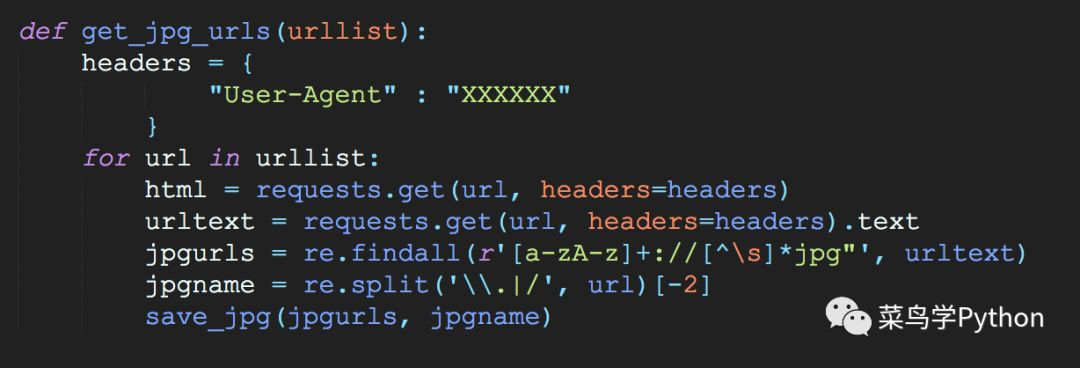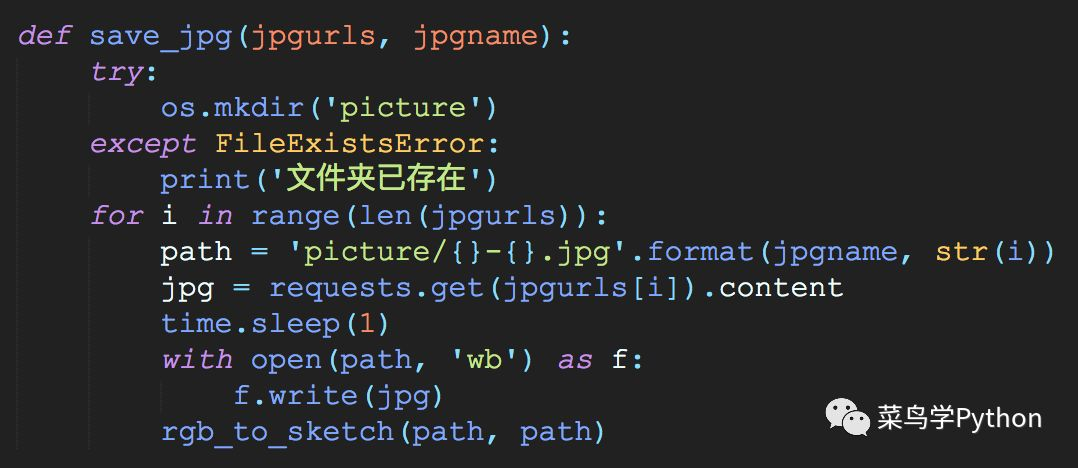``````def main():
idlist = ['id1', 'id2']
urllist = ['http://www.waxjj.cn/'+x+'.html' for x in idlist]
jpgurls = get_jpg_urls(urllist)``````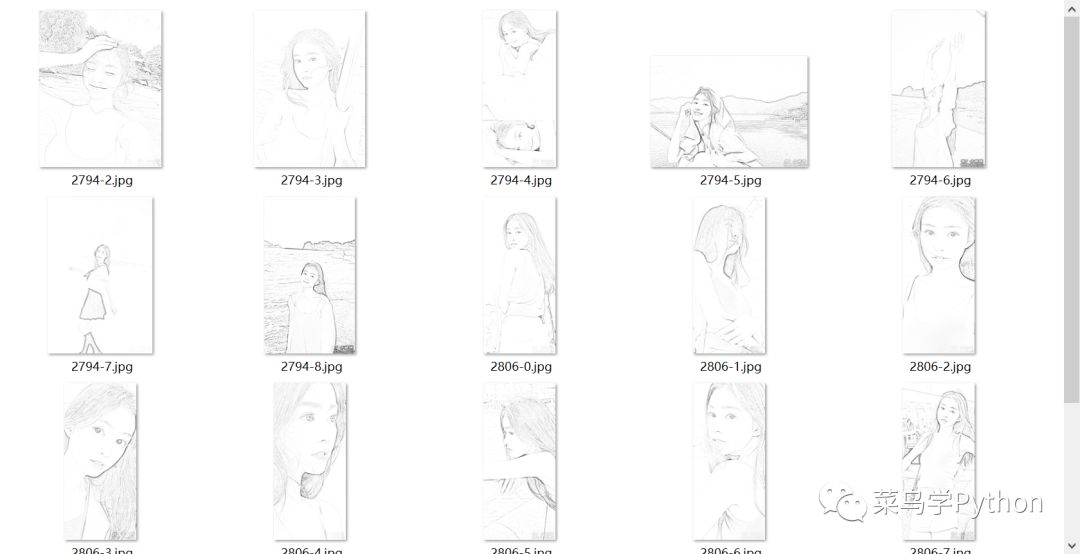https://www.cnblogs.com/7758520lzy/p/11976703.html

「点点赞赏，手留余香」

还没有人赞赏，快来当第一个赞赏的人吧！

Python
0 条回复 A 作者 M 管理员
所有的伟大，都源于一个勇敢的开始！